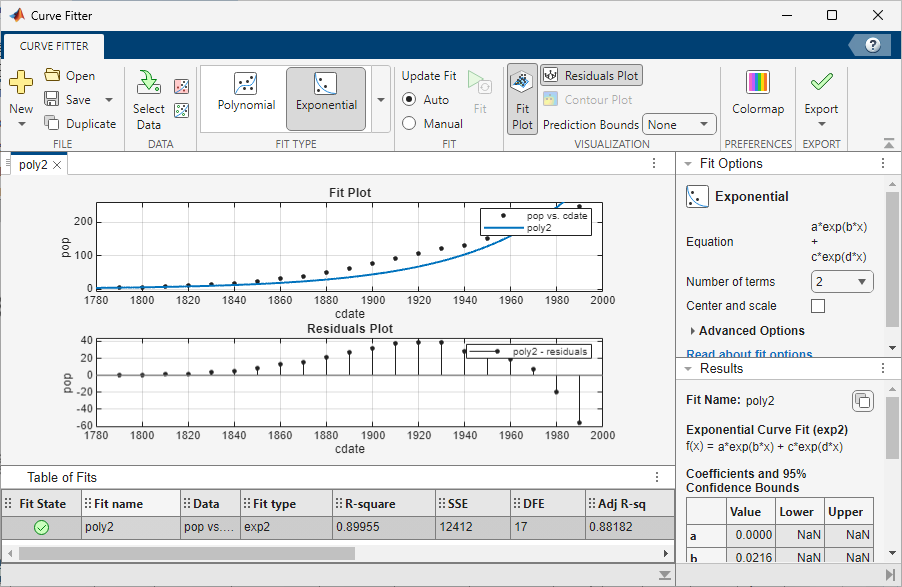Documentation

This is machine translation

Mouseover text to see original. Click the button below to return to the English version of the page.

Curve Fitting

Fit curves and surfaces to data

Description

The Curve Fitting app provides a flexible interface where you can interactively fit curves and surfaces to data and view plots.

You can:

• Create, plot, and compare multiple fits.

• Use linear or nonlinear regression, interpolation, smoothing, and custom equations.

• View goodness-of-fit statistics, display confidence intervals and residuals, remove outliers and assess fits with validation data.

• Automatically generate code to fit and plot curves and surfaces, or export fits to the workspace for further analysis.Open the Curve Fitting App

• MATLAB® Toolstrip: On the Apps tab, under Math, Statistics and Optimization, click the app icon.

• MATLAB command prompt: Enter `cftool`.

Programmatic Use

expand all

`cftool` opens Curve Fitting app or brings focus to the app if it is already open.

`cftool( x, y )` creates a curve fit to `x` input and `y` output. `x` and `y` must be numeric, have two or more elements, and have the same number of elements. `cftool` opens Curve Fitting app if necessary.

`cftool( x, y, z )` creates a surface fit to `x` and `y` inputs and `z` output. `x`, `y`, and `z` must be numeric, have two or more elements, and have compatible sizes. Sizes are compatible if `x`, `y`, and `z` all have the same number of elements or `x` and `y` are vectors, `z` is a 2D matrix, `length(x ) = n`, and `length(y) = m` where `[m,n] = size(z)`. `cftool` opens Curve Fitting app if necessary.

`cftool( x, y, [], w )` creates a curve fit with weights `w`. `w` must be numeric and have the same number of elements as `x` and `y`.

`cftool( x, y, z, w )` creates a surface fit with weights `w`. `w` must be numeric and have the same number of elements as `z`.

`cftool( filename )` loads the Curve Fitting session in `filename` into Curve Fitting app. The `filename` must have the extension `.sfit`.